# Weather Monitoring And WeatherForeCasting

This Project Aims Weather Monitoring,predicting and Analyzing using DHT11 sensor and ThingSpeak along with Algorithms.

ExpertFull instructions provided5 hours250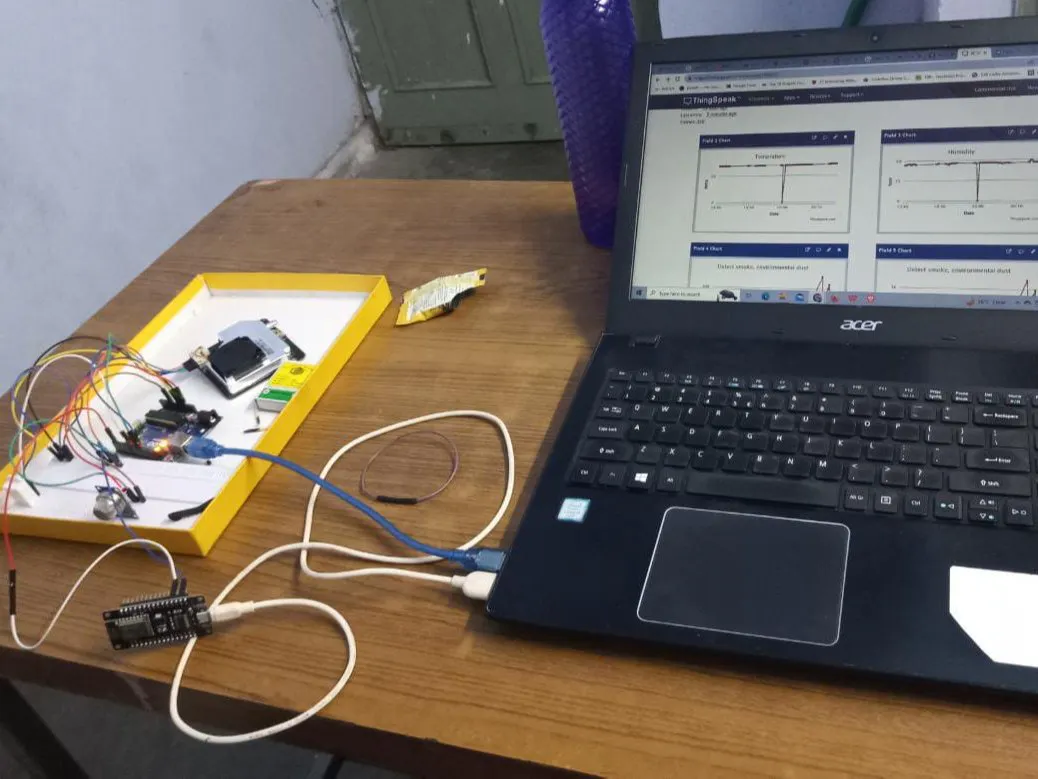## Things used in this project

### Hardware componentsArduino UNO
×1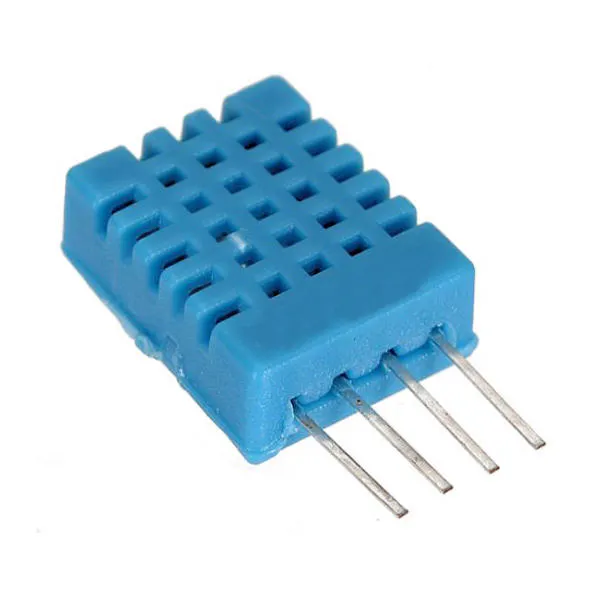DHT11 Temperature & Humidity Sensor (4 pins)
×1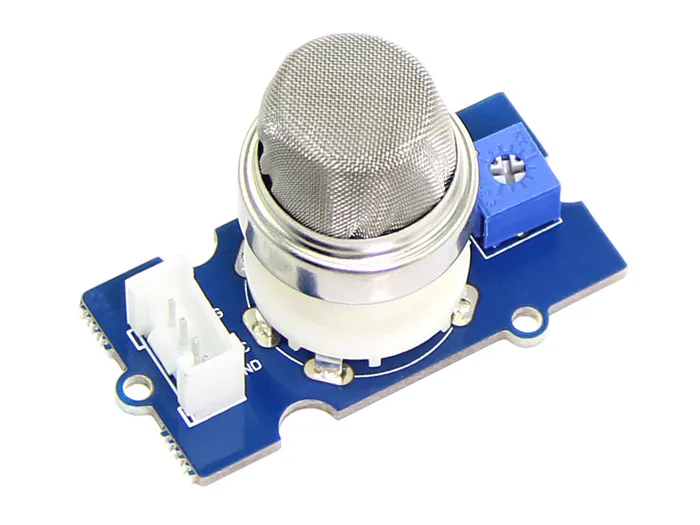Seeed Studio Grove - Gas Sensor(MQ2)
×1
 Nova PM Sensor SDS011 High Precision Laser pm2.5 Air Quality Detection Sensor
×1×1
 Male/Male Jumper Wires
×1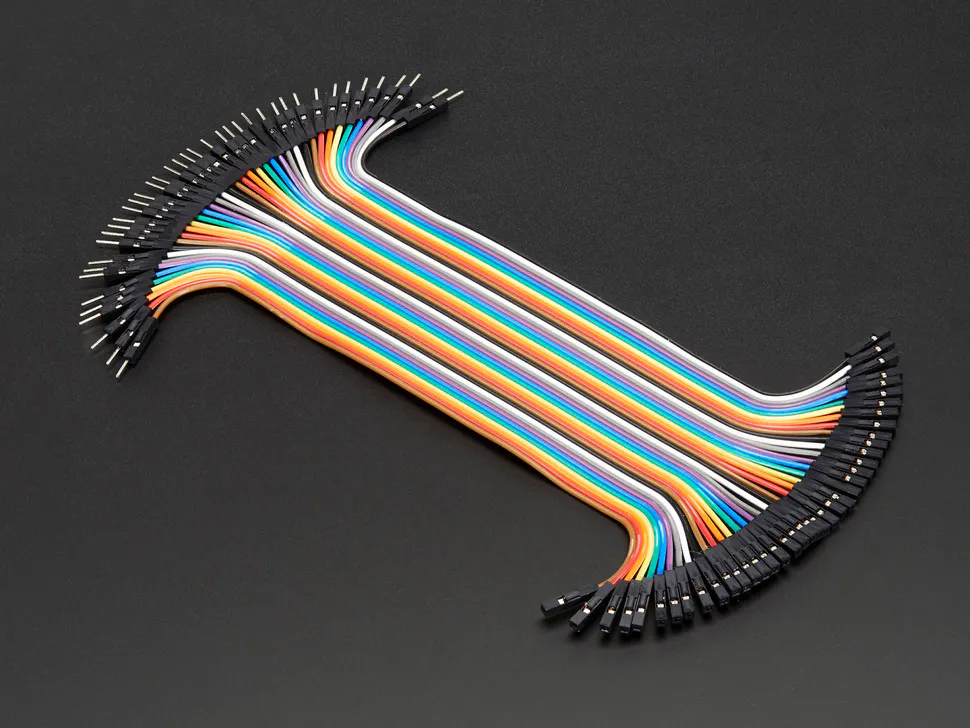Male/Female Jumper Wires
×1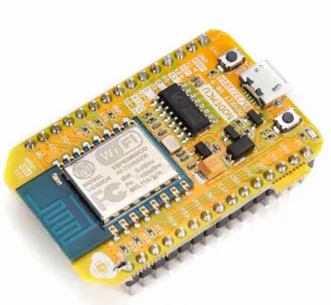NodeMCU ESP8266 Breakout Board
×1

### Software apps and online servicesThingSpeak APIMicrosoft Visual Studio Code Extension for ArduinoArduino IDE

## Schematics

### Systematic Diagram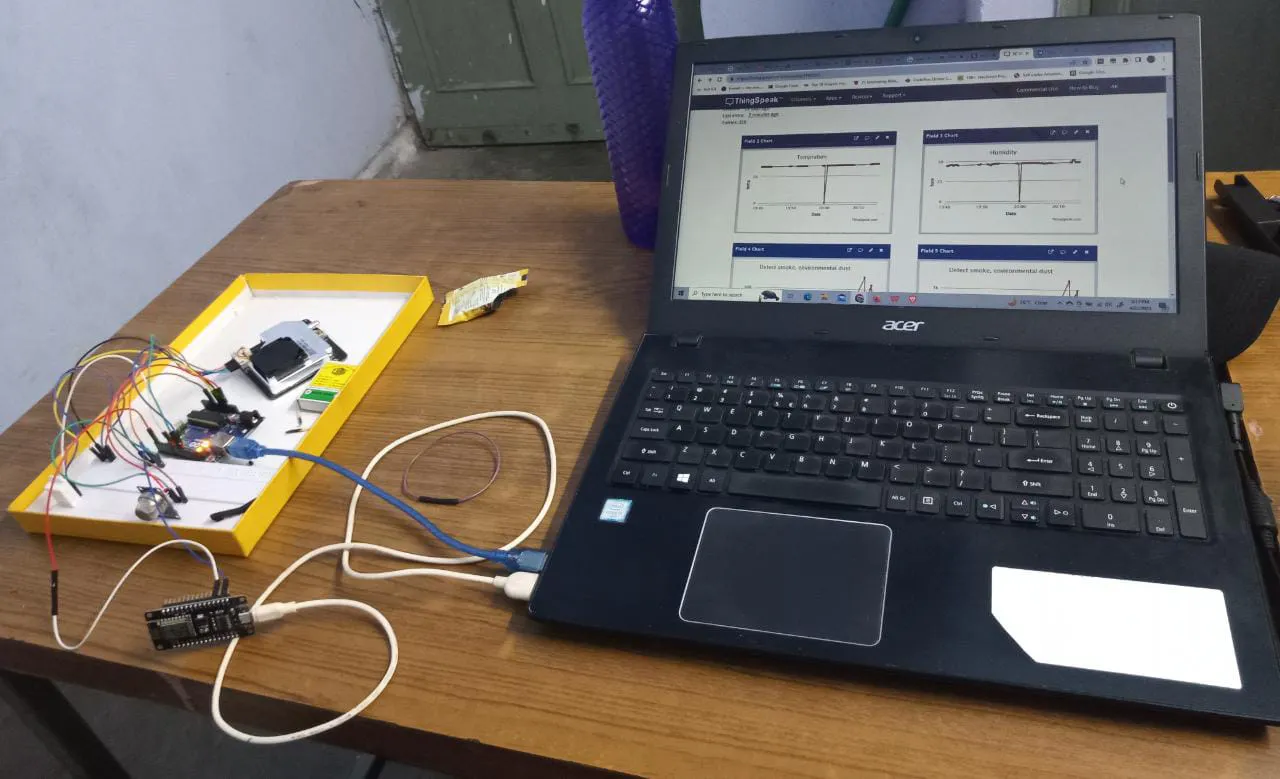### ThinkSpeak And Diagram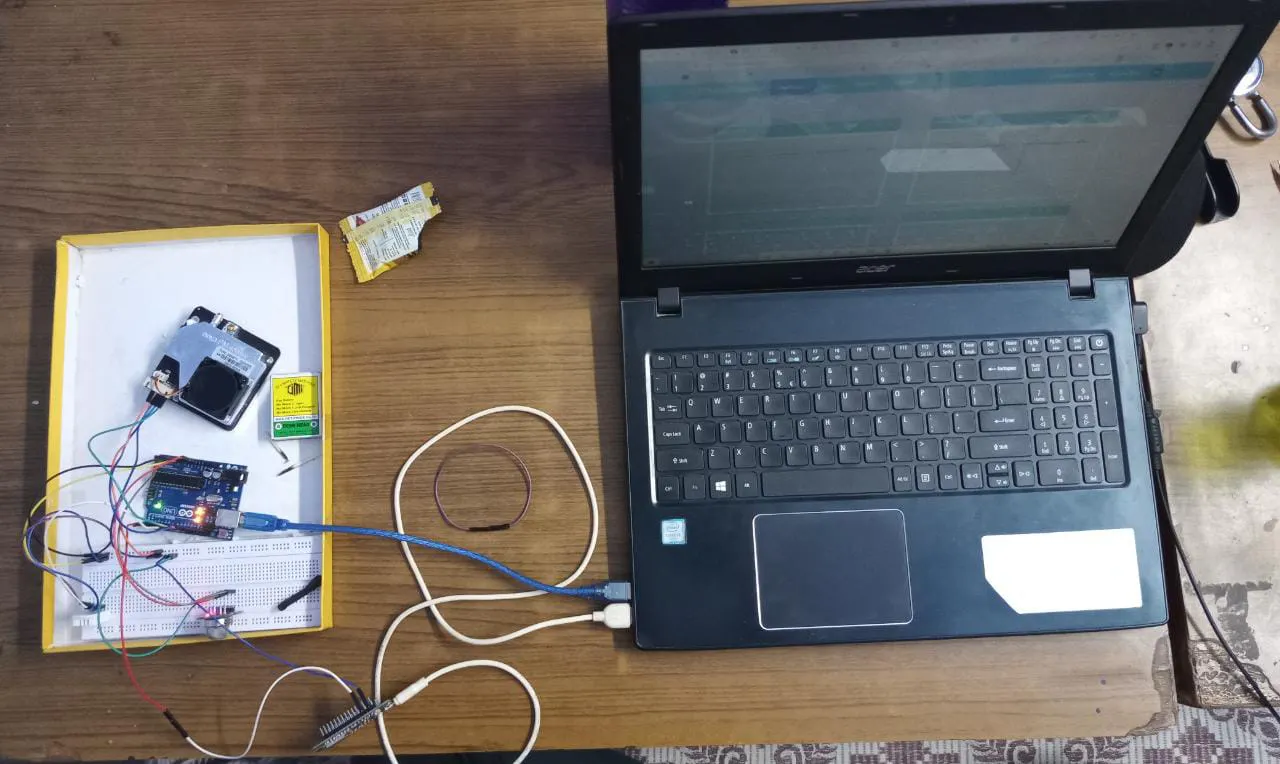### Diagram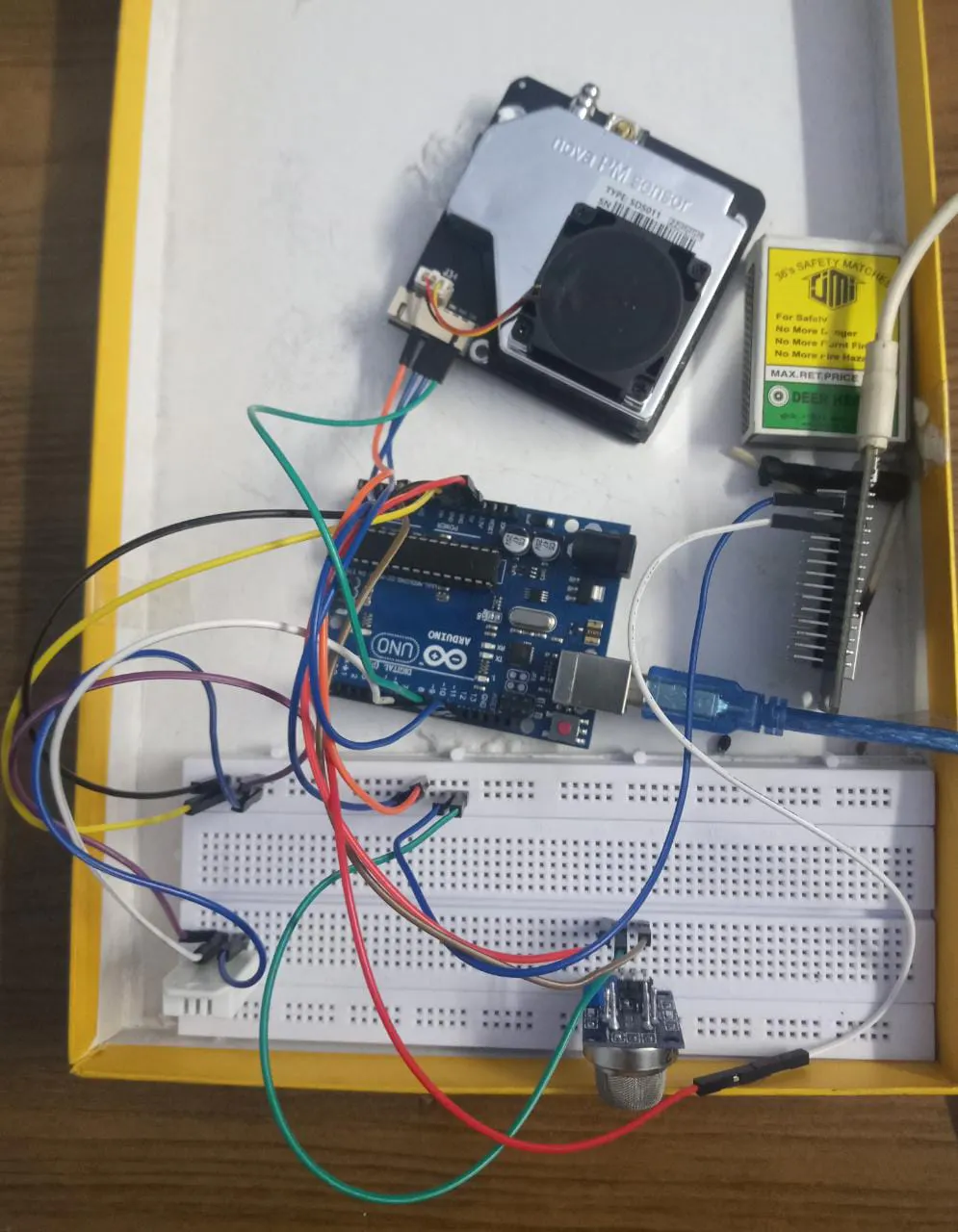## Code

### Arduino Code

Arduino
This Code is for Arduino
```//sender arduino

#include "DHT.h"
#define DHTPIN 8
#include <MQ2.h>
#define DHTTYPE DHT22
DHT dht(DHTPIN,DHTTYPE);

//PM sensor init
#include "SdsDustSensor.h"
int rxPin = 11;
int txPin = 12;
SdsDustSensor sds(rxPin, txPin);
//pm end

//Gas configuration starts
int Analog_Input = A0;
int lpg, co, smoke;
MQ2 mq2(Analog_Input);
float Level;
//Gas configuration ends

void setup() {
// put your setup code here, to run once:
Serial.begin(9600);
dht.begin();
mq2.begin();
sds.begin();
}

void loop() {

if (pm.isOk()) {
// Serial.print("PM2.5 = ");
// Serial.print(pm.pm25);
// Serial.print(", PM10 = ");
// Serial.println(pm.pm10);

// // if you want to just print the measured values, you can use toString() method as well
// Serial.println(pm.toString());
}

// put your main code here, to run repeatedly:
if(isnan(t) || isnan(h)){
//Serial.println("Data is not available");
return;
}

//gas

if (isnan(Level))

{
return;

}

//lpg = values;
//co = values;
//smoke = values;

//gas end
Serial.print(t);
Serial.print(",");
Serial.print(h);
Serial.print(",");

Serial.print(pm.pm25);
Serial.print(",");
Serial.print(pm.pm10);
Serial.print(",");

Serial.print(Level);
Serial.print(",");

Serial.println();

delay(10000);
}
```

### NodeMCU Code

C/C++
This Code for the NodeCMU WiFi Module
```//receiver arduino
#include <iostream>
#include<ESP8266WiFi.h>
#include<WiFiClient.h>
#include<ESP8266HTTPClient.h>

String URL ="http://api.thingspeak.com/update?api_key=LG44GXA088QY9060&field2=";

//float t,h,pm25,pm10;
void setup() {
// put your setup code here, to run once:
Serial.begin(9600);

//wifi
WiFi.disconnect();
delay(2000);
Serial.print("Start connection");

while((!(WiFi.status()== WL_CONNECTED))){
delay(200);
Serial.print("..");
}
Serial.println("Connected");

}

void loop() {
// put your main code here, to run repeatedly:
if(Serial.available()>0)
{
//Serial.println(data);
// int comma=data.indexOf(',');
// if(comma!=-1)
// {
//   float t=data.substring(0,comma).toFloat();
//   float h=data.substring(comma+1).toFloat();
//   Serial.print(t);
//   Serial.println(h);

// }
splitstr(data);

}

}

void splitstr(String data){
String  str = data;
String strs;
int StringCount = 0;

//Serial.println(str);

// Split the string into substrings
while (str.length() > 0)
{
int index = str.indexOf(',');
if (index == -1) // No space found
{
strs[StringCount++] = str;
break;
}
else
{
strs[StringCount++] = str.substring(0, index);
str = str.substring(index+1);
}
}
float arr;
// Show the resulting substrings
for (int i = 0; i < StringCount; i++)
{
//Serial.print(i);
//Serial.print(": \"");
if(strs[i].toFloat()!=0.0)
{
Serial.println((strs[i]).toFloat());
arr[i] = (strs[i]).toFloat();

}

//Serial.println("\"");
}
senddata(arr,arr,arr,arr,arr);

}

void senddata(float t, float h, float pm10, float pm20, float l)
{
WiFiClient client;
HTTPClient http;
String newUrl=URL+String(t)+"&field3="+String(h)+"&field4="+String(pm10)+"&field5="+String(pm20)+"&field6="+String(l);
http.begin(client,newUrl);
int responsecode=http.GET();
String data=http.getString();
Serial.println(data);
http.end();

}
```

### Algorithm Code

Python
This Code is for Training And Analysing the data ,further predicting the weather situation of upcoming Days
```import pandas as pd
import numpy as np
import matplotlib.pyplot as plt
import seaborn as sns
import plotly.express as px

print(data.describe())

print(data.info())

figure = px.line(data, x="date",
y="meantemp",
title='Mean Temperature in Delhi Over the Years')
figure.show()

figure = px.line(data, x="date",
y="humidity",
title='Humidity in Delhi Over the Years')
figure.show()

figure = px.line(data, x="date",
y="wind_speed",
title='Wind Speed in Delhi Over the Years')
figure.show()

figure = px.scatter(data_frame = data, x="humidity",
y="meantemp", size="meantemp",
trendline="ols",
title = "Relationship Between Temperature and Humidity")
figure.show()

forecast_data = data.rename(columns = {"date": "ds",
"meantemp": "y"})
print(forecast_data)

#Finally facebook prophet code to get upcoming days Weather Condition

from prophet import Prophet
from prophet.plot import plot_plotly, plot_components_plotly
model = Prophet()
model.fit(forecast_data)
forecasts = model.make_future_dataframe(periods=365)
predictions = model.predict(forecasts)
plot_plotly(model, predictions)
```